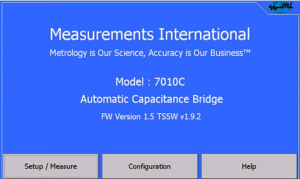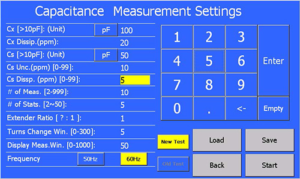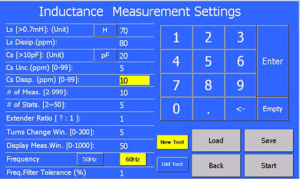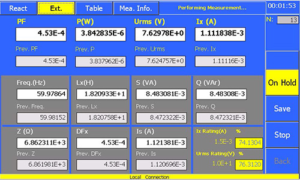## 7010C High-Voltage, Capacitance, Inductance, Tan Delta Bridge

• Automatic Self-Balancing
• Current Comparator Technology
• Range Extension Option with 7020
• Overall Ratio Accuracy < 15 ppm
• Low and High-Voltage Capacitance Measurements
• Potential Transformer Calibration
• Inductance Measurements
• Reactor Loss Measurements
Features & Benefits

The 7010C is a microprocessor controlled, current comparator based, automated capacitance bridge with metrology capabilities. However, it can also be used to measure inductance, reactor loss, AC resistance and the calibration of MI CTs. When used to measure capacitance the ratio of two capacitors (Cx/Cs) or the value of the capacitor Cx in capacitance units (pF to uF) or both are displayed along with the dissipation reading of the measurement. The minimal voltage that can be applied is 100 V when measuring a 1000 pF capacitor. To display the value of Cx, the value of the reference capacitor Cs must be known and entered through the touch screen before measurements start.

When it is used to measure inductance it displays either the ratio of 2 reactances (Xl/Xc, where Xl is the reactance of Lx, and Xc is the reactance of Cs) or the value of an unknown inductor in (mH to H). When measuring reactor loss, it displays inductance and various calculated parameters, such as power, power factor, input voltage and frequency. To display the value of L the value of the reference capacitor Cs must be known and entered through the touch screen before measurements start.

A large touch screen display presents relevant measuring quantities such as capacitance (Cx) and dissipation factor (Tan δ). Easy to use touch screen menus allows the operator to set up the measurements including the number of readings for statistical analysis of uncertainty calculations at the 95 % (2 s) level. The touch screen display can display a table of values. All measured parameters related to capacitance and inductance measurements can be transmitted over the IEEE-488 interface for storage to a computer. The 7010C measures and displays the current through the standard capacitor (Cs) and the applied input voltage and frequency of measurement. A USB slot is provided on the front panel for saving measurement data and parameters.

The principle of the 7010C is based on the two-stage-compensated current comparator. The automatic self-balancing feature facilitates the use of the bridge for accurate load loss measurements of large high-voltage inductive loads. This is a major advantage over the manual capacitance bridges which have difficulty in following frequency changes.

The model 7010C has the following ratios: 1:1, 2:1, 5:1, 10:1, 20:1, 50:1, 100:1, 200:1, 500:1 and 1000:1 with an overall accuracy of < 15 ppm in magnitude and 1 % of reading ± 10 ppm in dissipation. The dissipation (loss tangent) has a range of 0 to 10 % with a resolution of 1 ppm making it ideal for both low and high-voltage applications.

To accommodate capacitance ratios larger than 1000:1 an additional two-stage range extender, model 7020, may be added to increase the ratio to 2,000,000:1. All connections are made on the rear of the instrument. The effect of lead length on the measurement accuracy has been reduced by means of a built-in lead compensation circuit. An optional shielded rack on castors is available for portability on the test floor.

Applications for the 7010C include:

 Shunt Reactor Loss Measurements Power Transformer Measurements Calibration of Potential Transformers Calibration of Low-Voltage Std. Capacitors Calibration of High-Voltage Dividers Calibration of High-Voltage Power Capacitors Inductance Measurements Measurement of Low Loss, High-Voltage Power Loss Tangent Measurements to 10 % Corona Loss Measurements Insulator and Dielectric Testing

Capacitance Measurement and Calibration
The 7010C is capable of performing both 2 and 3 terminal capacitance measurements. Three terminal measurements (lead compensation) are typically performed when very large capacitors are to be measured to remove the lead impedance. For capacitance measurements the 7010C has a maximum ratio of 1000:1 for measurement of capacitance and a Cs range of 10 pF to 10,000 pF. To extend the ratio of the 7010C an additional range extender model 7020 with a ratio of up to 2000:1 can be added extending the ratio to 2,000,000:1. A large touch screen display on the 7010C is used to setup and display the measurements. Several high-voltage capacitors are also available ranging from 50 kV up to 800 kV with values of 50 pF and 100 pF.Voltage Transformer Calibration

Voltage transformers can be calibrated using the 7010C and two high-voltage standard capacitors. The ratio of the capacitors is first measured at the voltage that the transformer is measured at. The high-voltage supply is then used to feed both the voltage transformer and the two high-voltage standard capacitors. During the calibration of the voltage transformer the two high-voltage capacitors are interchanged and the voltage ratio can be calculated and the dissipation reading on the 7010C is the loss of the voltage transformer. Below table shows possible combination of ratios and capacitors but not limited to these values.

 Transformer Ratio Cs1 Cs2 Max Cs2 Volts Bridge Ratio 1 Bridge Ratio 2 1 1000 pF 1000 pF 26 kV 1 1 100 1000 pF 1000 pF 26 kV 1 100 10 1000 pF 100 pF 260 kV 10 1 1000 1000 pF 100 pF 260 kV 10 100 100 5000 pF 50 pF 520 kV 100 1 10000 5000 pF 50 pF 520 kV 100 100

Note: The same specifications for accuracy of the 7010C Capacitance Bridge apply to each of the two measurements required for voltage transformers. Any voltage coefficient of the Cs1 and Cs2 capacitor should be taken into account when calculating the voltage ratio.

Inductance MeasurementThe Capacitance Bridge is capable of measuring inductors by automatically reversing the primary winding. This reactive component of current when reversed is in phase with the current through the standard capacitor and a bridge balance can be obtained with the display reading in terms of equivalent inductance ratio.

Note: The accuracy of the bridge in the measurement of capacitance ratio does not depend essentially on the accuracy of the frequency. However, in measuring inductance, the frequency of the supply can be the dominating factor.

The 7010C handles frequency changes by measuring the frequency and updating the display. Several transformer manufacturers use the MI 7010C, 7020 and a MI high-voltage capacitor combination for measuring inductance and reactor losses. Systems as high as 600 kV have been built. The range of the 7010C can be extended for the measurement of inductance using the 7020 Two-Stage Compensated Current Transformer which has a single ratio up to 2000:1. The combined ratio of the 7010C and 7020 is 2,000,000:1. See inductance measurement range table for a complete range of inductance measurements.

Inductance Measurement Range
 Capacitor Cs (pF) Max Voltage  @ 50 Hz (V) Bridge Ratio Extender Ratio Max Inductance (H) Max Inductor Current (A) 10000 3180 723 2 0.7 14.46 10000 3180 723 20 0.07 144.6 10000 3180 723 200 0.007 1446 10000 3180 723 2000 0.0007 14460 1000 31800 723 2 7 14.46 1000 31800 723 20 0.7 144.6 1000 31800 723 200 0.07 1446 1000 31800 723 2000 0.007 14460 100 318000 723 2 70 14.46 100 318000 723 20 7 144.6 100 318000 723 200 0.7 1446 100 318000 723 2000 0.07 14460 50 636000 723 2 140 14.46 50 636000 723 20 14 144.6 50 636000 723 200 1.4 1446 50 636000 723 2000 0.14 14460 Capacitor Cs (pF) Max Voltage  @ 60 Hz (V) Bridge Ratio Extender Ratio Max Inductance (H) Max Inductor Current (A) 10000 2650 1005 1 0.7 10.04 10000 2650 1005 10 0.07 100.4 10000 2650 1005 100 0.007 1004 10000 2650 1005 1000 0.0007 10041 1000 26500 1005 1 7 10.04 1000 26500 1005 10 0.7 100.4 1000 26500 1005 100 0.07 1004 1000 26500 1005 1000 0.007 10041 1000 26500 1005 2000 0.0035 20083 100 265000 1005 1 70 10.04 100 265000 1005 10 7 100.4 100 265000 1005 100 0.7 1004 100 265000 1005 1000 0.07 10041 100 265000 1005 2000 0.035 20083 50 530000 1005 1 140 10.04 50 530000 1005 10 14 100.4 50 530000 1005 100 1.4 1004

Note: If other Inductance Measurement Ranges are required, contact Measurements International

Touch Screen Operation

When 7010C power switch is turned on – the touch screen will automatically load the program and the bridge will perform initialization routines, calibrate its A/D converters, etc.

From the touch screen panel it is possible to fully operate the bridge performing the capacitance, inductance and reactor loss measurements.

For each measurement the user will be prompted to enter parameter setting page.Specifications
 Capacitance Range Cs: 10 pF to 10,000 pF Cx: 10 pF to 10,000,000 pF (10 µF) Capacitive Ratio 1:1 to 1000:1 Ns: 0 to 1.11110 in steps of 0.000001 Nx: 1 to 1000 in steps of 1, 2, 5 Primary Current 10 Amp Maximum Secondary (Cs) Current Range 40 µA to 10 mA Dissipation Factor Range 0 to 10 % in steps of 0.000001 Inductance Range 700 µH to 700000 H (Q factor > 10) Test Frequencies 50 and 60 Hz Accuracy Ratio: ± 15 ppm for all Cx Ratios Loss Angle ± 1 % of Reading ± 10 ppm Display 7-inch Screen Display Warm Up Time < 5 Minutes to Full Rated Accuracy Operating Environment 18 to 34 °C, 10 to 80 % RH Operating Power 100, 120, 220, 240 V – 50/60 Hz